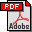Two theorems on the parallel construction of convex hulls Author: Jyrki Katajainen Publication: Report 93/23, Department of Computer Science, University of Copenhagen (1993), 10 pp. Full text:PDF (166 kB) Abstract: The parallel complexity of the problem of constructing the convex hull of a sorted planar point set is studied. For any point p in the plane, let x(p) and y(p) denote the x- and y-coordinate of p. A planar point set S = {p1, p2, …, pN} is said to be x-sorted if the points of S are given by increasing x-coordinate, i.e., x(pi) ≤ x(pi+1) for all i ∈ {1, 2, …, N−1}. The following two results are proved: Given an x-sorted set S of N points, the convex hull of S can be found in O(log N) time and O(N) space with ⌈N / log N⌉ processors on an EREW PRAM. Given an x-sorted set S of N points, a padded representation of the convex hull of S can be computed in O(loglog N) time and O(N) space with ⌈N / loglog N⌉ processors on a WEAK CRCW PRAM. (If the number of points in the convex hull is h, then the full representation of the convex hull is output in an array of size O(min{h1+ε, N}), for any fixed ε >0.) It is also shown that these algorithms are asymptotically fastest possible in their respective machine model if we insist on cost-optimality, i.e., that the product of the time complexity and the number of processors used is linear. BibLATEX: ```@report{Kat1993R, author = {Jyrki Katajainen}, title = {Two theorems on the parallel construction of convex hulls}, type = {Report}, number = {93/23}, institution = {Department of Computer Science, University of Copenhagen}, year = {1993}, pagetotal = {10}, } ```